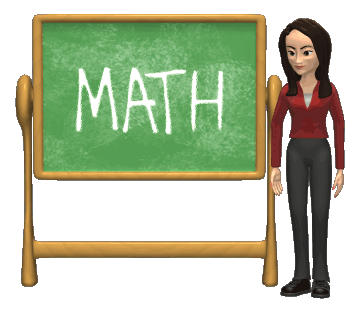# IIT JEE Mathematics Syllabus 2019The IIT JEE syllabus for mathematics has been broadly described below in the tabular format. The candidates preparing for the upcoming IIT JEE examination 2019 need to focus on each and every section to score good marks in the examinations. Enlisted is the recommended syllabus of Mathematics by CBSE for the JEE Main 2019 Examination.

## The IIT JEE Mathematics Syllabus is as under

 Algebra Algebra of complex numbers, addition, multiplication, conjugation, polar representation, properties of modulus and principal argument, triangle inequality, cube roots of unity, geometric interpretations. Quadratic equations with real coefficients, relations between roots and coefficients, formation of quadratic equations with given roots, symmetric functions of roots. Arithmetic, geometric and harmonic progressions, arithmetic, geometric and harmonic means, sums of finite arithmetic and geometric progressions, infinite geometric series, sums of squares and cubes of the first n natural numbers. Logarithms and their properties. Permutations and combinations, Binomial theorem for a positive integral index, properties of binomial coefficients. Matrices as a rectangular array of real numbers, equality of matrices, addition, multiplication by a scalar and product of matrices, transpose of a matrix, determinant of a square matrix of order up to three, inverse of a square matrix of order up to three, properties of these matrix operations, diagonal, symmetric and skew-symmetric matrices and their properties, solutions of simultaneous linear equations in two or three variables. Addition and multiplication rules of probability, conditional probability, Bayes Theorem, independence of events, computation of probability of events using permutations and combinations. Trigonometry Trigonometric functions, their periodicity and graphs, addition and subtraction formulae, formulae involving multiple and sub-multiple angles, general solution of trigonometric equations. Analytical Geometry Relations between sides and angles of a triangle, sine rule, cosine rule, half-angle formula and the area of a triangle, inverse trigonometric functions (principal value only). Two dimensions Cartesian coordinates, distance between two points, section formulae, shift of origin Equation of a straight line in various forms, angle between two lines, distance of a point from a line; Lines through the point of intersection of two given lines, equation of the bisector of the angle between two lines, concurrency of lines; Centroid, orthocentre, incentre and circumcentre of a triangle. Equation of a circle in various forms, equations of tangent, normal and chord. Parametric equations of a circle, intersection of a circle with a straight line or a circle, equation of a circle through the points of intersection of two circles and those of a circle and a straight line. Equations of a parabola, ellipse and hyperbola in standard form, their foci, directrices and eccentricity, parametric equations, equations of tangent and normal. Locus Problems. Three dimensions Direction cosines and direction ratios, equation of a straight line in space, equation of a plane, distance of a point from a plane. Differential calculus Real valued functions of a real variable, into, onto and one-to-one functions, sum, difference, product and quotient of two functions, composite functions, absolute value, polynomial, rational, trigonometric, exponential and logarithmic functions. Limit and continuity of a function, limit and continuity of the sum, difference, product and quotient of two functions, L’Hospital rule of evaluation of limits of functions. Even and odd functions, inverse of a function, continuity of composite functions, intermediate value property of continuous functions. Derivative of a function, derivative of the sum, difference, product and quotient of two functions, chain rule, derivatives of polynomial, rational, trigonometric, inverse trigonometric, exponential and logarithmic functions. Derivatives of implicit functions, derivatives up to order two, geometrical interpretation of the derivative, tangents and normals, increasing and decreasing functions, maximum and minimum values of a function, Rolle’s Theorem and Lagrange’s Mean Value Theorem. Integral calculus Integration as the inverse process of differentiation, indefinite integrals of standard functions, definite integrals and their properties, Fundamental Theorem of Integral Calculus. Integration by parts, integration by the methods of substitution and partial fractions, application of definite integrals to the determination of areas involving simple curves. Formation of ordinary differential equations, solution of homogeneous differential equations, separation of variables method, linear first order differential equations. Vectors Addition of vectors, scalar multiplication, dot and cross products, scalar triple products and their geometrical interpretations.

## JEE Main 2019 –Related Links

JEE Main Chemistry Syllabus

JEE Main Physics Syllabus

JEE Main Tips & Tricks